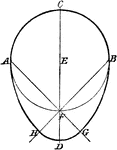### Construction of Oval

Illustration used to construct and oval when given the width.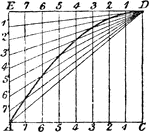### Construction of Parabola Given Base and Height

Illustration used to draw a parabola when given the base and height.### Construction Of A Parabola

An illustration showing how to construct a parabola by plotting. "Having given the axis, vertex, and…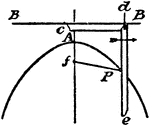### Construction Of A Parabola

An illustration showing how to construct a parabola using a pencil and a string. "Having given the two…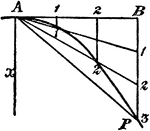### Construction Of A Parabola

An illustration showing how to construct a parabola. "Given the vertex A, axis x, and a point P. Draw…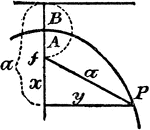### Construction Of A Parabola

An illustration showing how to construct a parabola. "Given the axis of ordinate B, and vertex A. Take…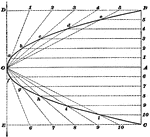### Construction Of Parabola

Illustration used to show how to draw a parabola when the axis and longest double ordinate is given.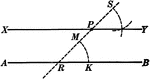### Construction Of A Parallel Line

Illustration of the construction used to create a line parallel to a given line.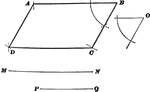### Construction Of Parallelogram

Illustration used to show how to draw a parallelogram when given the sides and one of the angles.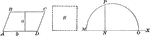### Parallelogram Used to Construct Equivalent Square

Illustration of a how to construct a square equivalent to a given parallelogram.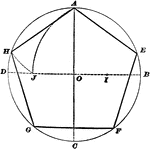### Construction Of Pentagon Inscribed In Circle

Illustration used to show how to inscribe a regular pentagon in a given circle.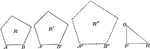### Pentagon Constructed to be Similar to Given Pentagons

Illustration of a how to construct a pentagon similar to two given pentagons.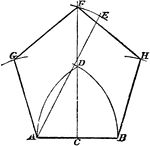### Construction of Regular Pentagon

Illustration used to construct a regular pentagon on a given line.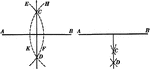### Construction Of A Perpendicular Bisector Of A Straight Line

Illustration of the construction used to make a perpendicular bisector of a straight line.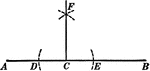### Construction Of A Perpendicular To A Straight Line

Illustration of the construction used to create a perpendicular to a straight line at a given point.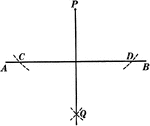### Construction Of A Perpendicular To A Straight Line

Illustration of the construction used to create a perpendicular to a straight line from a given point…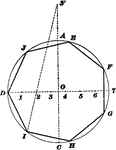### Construction Of Polygon Inscribed In Circle

Illustration used to show how to inscribe any regular polygon in a given circle.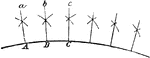### Construction of Radii of a Circle

Illustration used to draw lines which are radii of a circle where the center is inaccessible.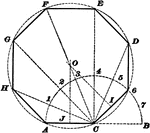### Construction Of Regular Polygon

Illustration used to show how to draw a regular polygon when given a side of the polygon.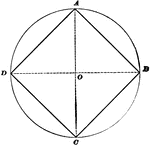### Construction Of Square Inscribed In Circle

Illustration used to show how to inscribe a square in a given circle.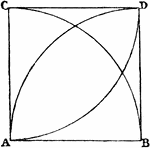### Construction of Square on Given Line

Illustration used to construct a square on a given line.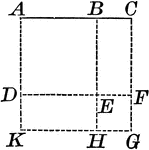### Square Constructed Upon the Sum of Two Straight Lines

Illustration of a square constructed upon the sum of two straight lines.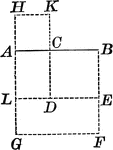### Square Constructed Upon Difference of Lines

Illustration of a square constructed upon the difference of two straight lines.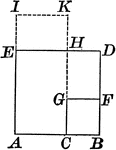### Square Constructed Upon Difference of Lines

Illustration of a square constructed upon the difference of two straight lines.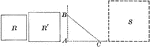### Square Constructed to be Equal to the Sum of Two Given Squares

Illustration of a how to construct a square equivalent to the sum of two given squares.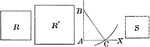### Square Constructed to be Equal to the Difference of Two Given Squares

Illustration of a how to construct a square equivalent to the difference of two given squares.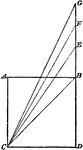### Construction of Multiple of a Square

Illustration used to construct a square that shall be a multiple of any given square.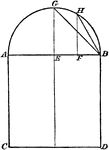### Construction of Proportional Square

Illustration used to construct a square that shall be in proportion to a given square.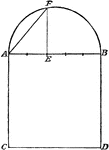### Construction of Proportional Square

Illustration used to construct a square that shall be in proportion to a given square.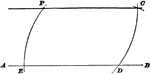### Parallel To Straight Line Construction

Illustration used to show how to draw a straight line parallel to a straight line through a given point.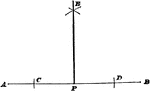### Perpendicular To Straight Line Construction

Illustration used to show how to draw a perpendicular to a straight line from a given point on the line…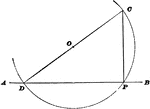### Perpendicular To Straight Line Construction

Illustration used to show how to draw a perpendicular to a straight line from a given point on the line…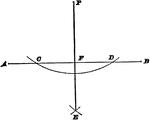### Perpendicular To Straight Line Construction

Illustration used to show how to draw a perpendicular to a straight line from a given point that is…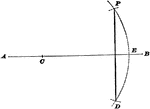### Perpendicular To Straight Line Construction

Illustration used to show how to draw a perpendicular to a straight line from a given point that is…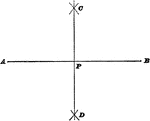### Bisect A Straight Line

Illustration used to show how to bisect a straight line.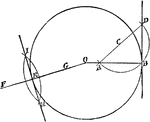### Construction of Tangent to a Circle

Illustration used to draw a tangent to a circle.### Construction of a Tangent to a Circle

Illustration used to show how "to construct a tangent to a circle from a point outside."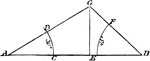### Construction of Triangle Given Angles and Base

Illustration used to construct a triangle given the length of the base and the two base angles.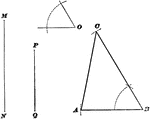### Construction Of Triangle

Illustration used to show how to draw a triangle when given two sides and the included angle of the…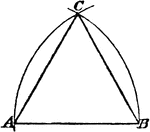### Construction of an Equilateral Triangle

Illustration used to construct an equilateral triangle on a given base.